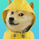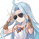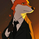# Computing The Linear Regression Using The WMA And SMA

1545 obejrzeń
Plot a linear regression channel through the last length closing prices, with the possibility to use another source as input. The line is fit by using linear combinations between the WMA and SMA thus providing both an interesting and efficient method. The results are the same as the one provided by the built-in linear regression , only the computation differ.

Settings

• length : Number of inputs to be used.
• src : Source input of the indicator.
• mult : Multiplication factor for the RMSE, determine the distance between the upper and lower level.

Usage

In technical analysis a linear regression can provide an estimate of the underlying trend in the price, this result can be extrapolated to have an estimate of the future evolution of the trend, while the upper and lower level can be used as support and resistance levels.

The slope of the fitted line indicates both the direction and strength of the trend, with a positive slope indicating an up-trending market while a negative slope indicates a down-trending market, a steeper line indicates a stronger trend.

We can see that the trend of the S&P500 in this chart is approximately linear, the upper and lower levels were previously tested and might return accurate support and resistance points in the future.

By using a linear regression we are making the following assumptions:

• The trend is linear or approximately linear.
• The cycle component has an approximately constant amplitude (this allows the upper and lower level to be more effective)
• The underlying trend will have the same evolution in the future

In the case where the growth of a trend is non-linear, we can use a logarithmic scale to have a linear representation of the trend.

Details

In a simple linear regression , we want to the slope and intercept parameters that minimize the sum of squared residuals between the data points and the fitted line

`intercept + x*slope`

Both the intercept and slope have a simple solution, you can find both in the calculations of the lsma , in fact, the last point of the lsma with period length is equal to the last point of a linear regression fitted through the same length data points. We have seen many times that the lsma is an FIR filter with a series of coefficients representing a linearly decaying function with the last coefficients having a negative value, as such we can calculate the lsma more easily by using a linear combination between a WMA and SMA: 3WMA - 2SMA, this linear combination gives us the last point of our linear regression , denoted point B.

Now we need the first point of our linear regression , by using the calculations of the lsma we get this point by using:

`intercept + (x-length+1)*slope`

If we get the impulse response of such lsma we get

In blue the impulse response of a standard lsma , in red the impulse response of the lsma using the previous calculation, we can see that both are the same with the exception that the red one appears as being time inverted, the first coefficients are negative values and as such we also have a linear operation involving the WMA and SMA but with inverted terms and different coefficients, therefore the first point of our linear regression , denoted point A, is given by 4SMA - 3WMA, we then only need to join these two points thanks to "line.new".

The levels are simply equal to the fitted line plus/minus the root mean squared error between the fitted line and the data points, right now we only have two points, we need to find all the points of the fitted line, as such we first need to find the slope, which can be calculated by diving the vertical distance between B and A (the rise) with the horizontal distance between B and A (the run), that is

`(A - B)/(length-1)`

Once done we can find each point of our line by using

`B + slope*i`

where i is the position of the point starting from B, i=0 give B since B + slope*0 = B, then we continue for every i, we then only need to sum the squared distance between each closing prices at position i and the point found at that same position, we divide by length-1 and take the square root of the result in order to have the RMSE.

In Summary

The following post as shown that it was possible to compute a linear regression by using a linear combination between the WMA and SMA , since both had extremely efficient computations (see link at the end of the post) we could have a calculation for the linear regression where the number of operations is independent of length.

This post took me eons to make because it's related to the lsma , and I am rarely short on words when it comes to anything related to the lsma . Thx to LucF for the feedback and everything.

« Je suis las des cruautés de mes semblables, qui ne sont pas mes pareils.

« Je prendrai l’essor et je m’envolerai vers la mer.

« Je connaîtrai le goût des brises du large. J’entendrai les grands cris de la tempête.

## KomentarzeI would not be wasting so much time figuring out "new maths" to get to my goal.
This method does not use STDEV and every time STDEV is kicked out of something I sleep better at night.
OdpowiedzTetsumaz
@Tetsumaz, Thx for your support, altho that's quite strange, stdev is pretty harmless in my opinion xD
OdpowiedzGreat work! Was wondering how to make an alert from this when price closes outside the regression channel. But not sure if it's possible since the plotted lines are extensions.
Odpowiedzjoshnomics